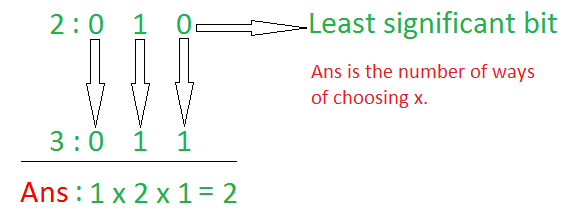# Count values whose Bitwise OR with A is equal to B

• Last Updated : 14 Jun, 2021

Given two integers A and B, the task is to count possible values of X that satisfies the condition A | X = B.
Note: | represents Bitwise OR operation.

Examples:

Input: A = 2, B = 3
Output: 2
Explanation:
Since, 2 | 1 = 3 and 2 | 3 = 3. Therefore, the possible values of x are 1 and 3.

Input: A = 5, B = 7
Output: 4

Naive Approach: The simplest approach to solve this problem is to iterate over the range [1, B] and check for every number, whether its Bitwise OR with A is equal to B. If the condition is satisfied, increment the count.
Time Complexity: O(b)
Auxiliary Space: O(1)

Efficient Approach: The above approach can be optimized based on the following observations:

• Convert the numbers A and B into their respective binary representations.
• Truth table of Bitwise OR operation:
1 | 1 = 1
1 | 0 = 1
0 | 1 = 1
0 | 0 = 0

• For each same-positioned bit, count number of ways the ith bit in A can be converted to the ith bit in B by performing Bitwise OR operation.
•Follow the steps below to solve the problem:

• Initialize a variable ans to store the required result.
• Traverse the bits of a and b simultaneously using the variable i
• If the ith bit of a is set and the ith bit of b is also set, then the ith bit of x can take 2 values, i.e, 0 or 1. Hence, multiply the ans by 2.
• If the ith bit of a is unset and the ith bit of b is set, then the ith bit of x can take only 1 value, i.e, 1. Hence, multiply the ans by 1.
• If the ith bit of a is set and the ith bit of b is unset, then no matter what the ith bit of x is, the ith bit of a can not be converted to ith bit of b. Hence, update ans to 0 and break out of the loop.
• Print the value of ans as the result

Below is the implementation of the above approach:

## C++

 `// C++ program for the above approach ` `#include ` `using` `namespace` `std; ` ` `  `// Function to count possible values of ` `// X whose Bitwise OR with A is equal to B ` `int` `numWays(``int` `a, ``int` `b) ` `{ ` ` `  `    ``// Store the required result ` `    ``int` `res = 1; ` ` `  `    ``// Iterate over all bits ` `    ``while` `(a && b) { ` ` `  `        ``// If i-th bit of a is set ` `        ``if` `((a & 1) == 1) { ` ` `  `            ``// If i-th bit of b is set ` `            ``if` `((b & 1) == 1) { ` ` `  `                ``// The i-th bit in x ` `                ``// can be both 1 and 0 ` `                ``res = res * 2; ` `            ``} ` `            ``else` `{ ` ` `  `                ``// a|x is equal to 1 ` `                ``// Therefore, it cannot ` `                ``// be converted to b ` `                ``return` `0; ` `            ``} ` `        ``} ` ` `  `        ``// Right shift a and b by 1 ` `        ``a = a >> 1; ` `        ``b = b >> 1; ` `    ``} ` ` `  `    ``return` `res; ` `} ` ` `  `// Driver Code ` `int` `main() ` `{ ` `    ``// Given Input ` `    ``int` `a = 2, b = 3; ` ` `  `    ``// Function Call ` `    ``cout << numWays(a, b); ` ` `  `    ``return` `0; ` `}`

Output:

```2
```

Time Complexity: O(max(log a, log b))
Auxiliary Space: O(1)

My Personal Notes arrow_drop_up
Recommended Articles
Page :